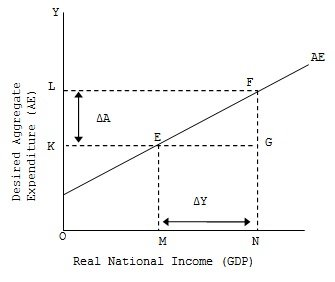Aggregate Spending

# Aggregate Spending Assignment Help

Home / Macroeconomics Assignment Help / Aggregate Spending

# Aggregate Spending

Aggregate spending function relates the volume of aggregate expenditure to various levels of national income It shows how much the desired aggregate expenditure would be at various levels of income. Other things being equal aggregate spending will be larger at higher income levels and smaller at tower levels. In terms of diagrammatic presentation, there will be movement along the same AE curve. This is shown in the diagram.As is clear from the above diagram, when real national income (GDP) goes up from OM to ON, aggregate expenditure increase from OK to OL. The increase in aggregate expenditure FG = KL or ΔA is due to an increase in national income EG = MN or ΔY. Thus, the aggregate expenditure (AE) curve remains the same and there is a movement from E to F on the same curve.

### Submit Homework

Submit your homework for a free quote